In this project we are going to design a 25V range digital voltmeter by using ATMEGA32A microcontroller. In ATMEGA, we are going to use 10bit ADC (Analog to Digital Converter) to build a digital voltmeter. Now the ADC in ATMEGA can not take a input more than +5V, so for getting a higher range we are going to use a voltage divider circuit.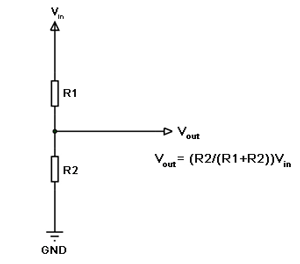Now important thing to note here is, the input taken by the controller for ADC conversion is as low as 50µAmp. This loading effect of resistance based voltage divider is important as the current drawn from Vout of voltage divider increases the error percentage, for now we need not worry about loading effect.

We are going to take two resistors and form a divider circuit so that for a 25Volts Vin, we get a 5Volt Vout. So all we have to do is multiply the Vout value with “5” in the program in order to get the real input voltage.

### Components Required

Hardware: ATMEGA32, Power supply (5v), AVR-ISP PROGRAMMER, JHD_162ALCD (16*2LCD), 100uF capacitor, 100nF capacitor (5 pieces), 10KΩ resistor, 2KΩresistor, 1KΩ pot or preset.

Software: Atmel studio 6.1, progisp or flash magic.

### Circuit Diagram and Working Explanation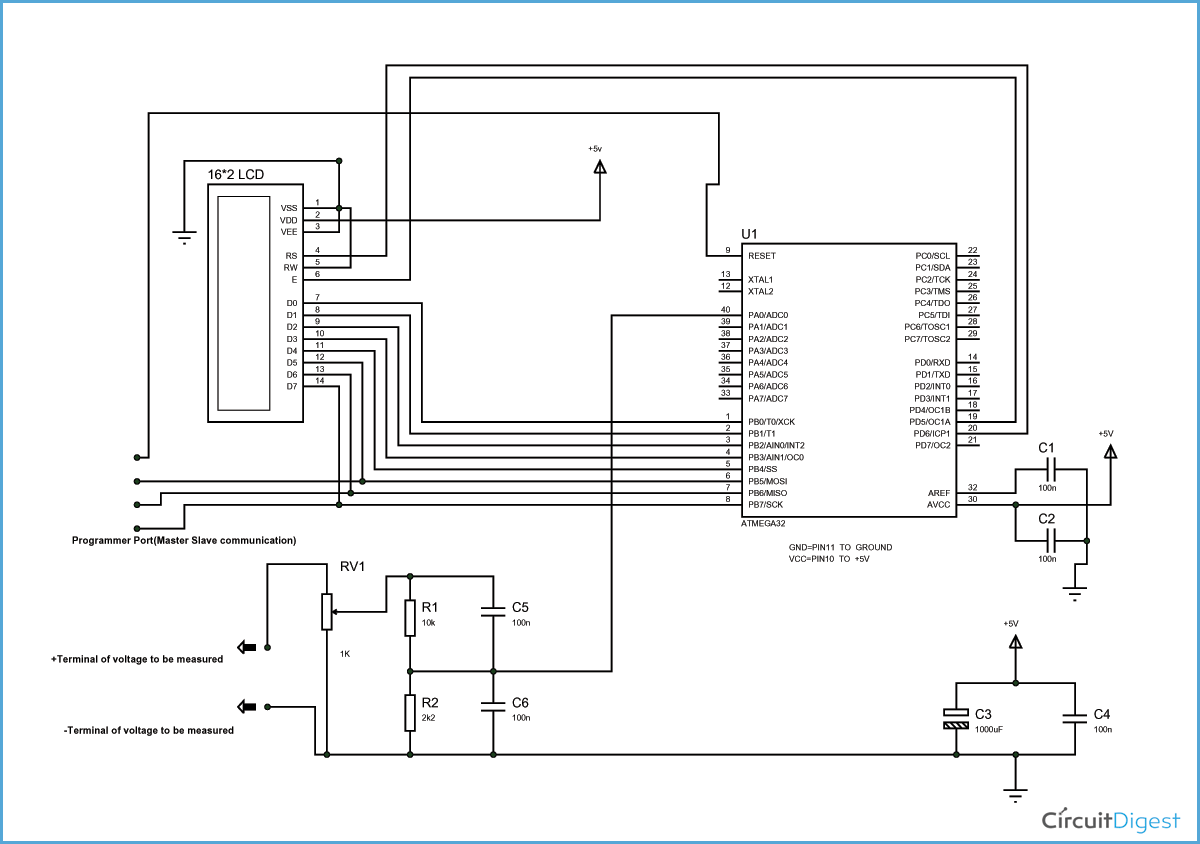The complete digital voltmeter circuit diagram is shown in the above figure. Here PORTB of ATMEGA32 is connected to data port of LCD. One should remember to disable the JTAG communication in PORTC ot ATMEGA by changing the fuse bytes, if one wants to use the PORTC as a normal communication port. In 16x2 LCD there are 16 pins total if there is a black light, if there is no back light there will be 14 pins. One can power or leave the back light pins. Now in the 14 pins there are 8 data pins (7-14 or D0-D7), 2 power supply pins (1&2 or VSS&VDD or gnd&+5v), 3rd pin for contrast control (VEE-controls how thick the characters should be shown), and 3 control pins (RS&RW&E). (Learn more about LCD in this tutorial: LCD Interfacing with AVR Microcontroller).

In the circuit, you can observe that I have only took two control pins , this gives the flexibility of better understanding, the contrast bit and READ/WRITE are not often used so they can be shorted to ground. This puts LCD in highest contrast and read mode. We just need to control ENABLE and RS pins to send characters and data accordingly.

The connections which are done for LCD are given below:

PIN1 or VSS to ground

PIN2 or VDD or VCC to +5v power

PIN3 or VEE to ground (gives maximum contrast best for a beginner)

PIN4 or RS (Register Selection) to PD6 of uC

PIN5 or RW (Read/Write) to ground (puts LCD in read mode eases the communication for user)

PIN6 or E (Enable) to PD5 of uC

PIN7 or D0 to PB0 of uC

PIN8 or D1 to PB1 of uC

PIN9 or D2 to PB2 of uC

PIN10 or D3 to PB3 of uC

PIN11 or D4 to PB4 of uC

PIN12 or D5 to PB5 of uC

PIN13 or D6 to PB6 of uC

PIN14 or D7 to PB7 of uC

In the circuit you can see that we have used 8bit communication (D0-D7) however this is not a compulsory, we can use 4bit communication (D4-D7) but with 4 bit communication program becomes a little bit complex. We are connecting 10 pins of LCD to microcontroller in which 8 pins are data pins and 2 pins for control.

The voltage across R2 (2.2KΩ) is not completely linear; it will be a noisy one. To filter out the noise, capacitors are placed across each resistor in the divider circuit as shown in the circuit diagram above.

The 1K pot here is to adjust the accuracy of ADC. In ATMEGA32A, we can give Analog input to any of eight channels of PORTA, it doesn’t matter which channel we choose as all are same, we are going to choose channel 0 or PIN0 of PORTA.

In ATMEGA32A, the ADC is of 10 bit resolution, so the controller can detect and sense a minimum change of Vref/2^10, so if the reference voltage is 5V, we get a digital output increment for every 5/2^10 = 5mV. So for every 5mV increment in the input we will have a increment of one at digital output.

Now we need to set the register of ADC based on the following terms:

1.First of all we need to enable the ADC feature in ADC.

2. The maximum input voltage for ADC conversion is +5V (5*(12.2/2.2) =27.7V; since R1 = 10KΩ and R2 = 2.2KΩ ). So we can set up maximum value or reference of ADC to 5V.

3. The controller has a trigger conversion feature, that means ADC conversion takes place only after an external trigger, since we don’t want that we need to set the registers for the ADC to run in continuous free running mode.

4. For any ADC, frequency of conversion (Analog value to Digital value) and accuracy of digital output are inversely proportional. So for better accuracy of digital output we need to choose lesser frequency. For lesser ADC clock we are setting the presale of ADC to maximum value (128). Since we are using the internal clock of 1MHZ, the clock of ADC will be (1000000/128).

These are the only four things we need to know to getting started with ADC.

All the above four features are set by two registers: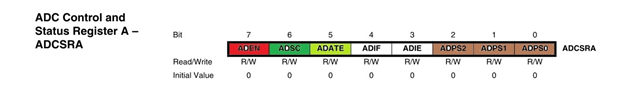RED (ADEN): This bit has to be set for enabling the ADC feature of ATMEGA.

BLUE(REFS1,REFS0): These two bits are used to set the reference voltage (or max input voltage we are going to give).  Since we want to have reference voltage 5V, REFS0 should be set, by the table.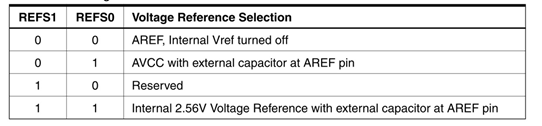LIGHT GREEN (ADATE): This bit must be set for the ADC to run continuously (free running mode).

PINK (MUX0-MUX4): These five bits are for telling the input channel. Since we are going to use ADC0 or PIN0, we need not set any bits as given in the table.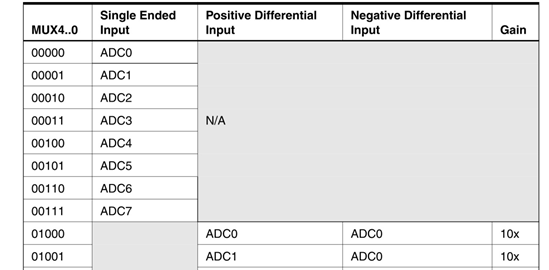BROWN (ADPS0-ADPS2): these three bits are for setting the prescalar for ADC. Sice we are using a prescalar of 128, we need to set all the three bits.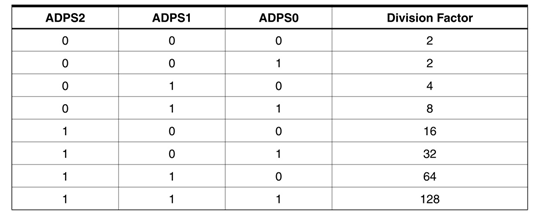DARK GREEN (ADSC): this bit set for the ADC to start conversion. This bit can be disabled in the program when we need to stop the conversion.

### Programming Explanation

Working of this digital voltmeter project is explained step by step in the below code.

Code

/*
* C code for Digital Voltmeter using ATmega32 Microcontroller
*/
#include <avr/io.h>
#define F_CPU 1000000
#include <util/delay.h>
#include <stdlib.h>

#define enable            5
#define registerselection 6

void send_a_command(unsigned char command);
void send_a_character(unsigned char character);
void send_a_string(char *string_of_characters);

int main(void)
{
DDRB = 0xFF;
DDRA = 0;
DDRD = 0xFF;
_delay_ms(50);

float voltage = 0;
char voltageshow ;

send_a_command(0x01); //Clear Screen 0x01 = 00000001
_delay_ms(50);
send_a_command(0x38);
_delay_ms(50);
send_a_command(0b00001111);
_delay_ms(50);

while(1)
{
send_a_string ("CIRCUIT DIGEST  ");
send_a_command(0x80 + 0x40 + 0);
send_a_string ("VOLTAGE=");
send_a_command(0x80 + 0x40 + 8);
dtostrf(voltage, 5, 2, voltageshow);
send_a_string(voltageshow);
send_a_string("V   ");
//dtostr(double precision value, width, precision, string that will store the numbers);
// Value is either a direct value plugged into this place, or a variable to contains a value.
//Width that is used with dtostrf is the number of characters in the number that includes the negative sign (-). For instance, if the number is -532.87, the width would be 7 including the negative sign and the decimal point.
//Precision is how many numbers would be after the decimal point in the dtostrf usage.
//Base is the maximum number of values per digit. For instance, 2 is for binary representation (2 possible values for each digit - 0 or 1); 10 is for the common human number system (10 possible values for each digit - 0, 1, 2, 3, 4, 5, 6, 7, 8, or 9); 16 is for hexadecimal where there are 16 possible values for each digit - 0, 1, 2, 3, 4, 5, 6, 7, 8, 9, A, B, C, D, E, or F. It should be understood that if the base is larger, then the alphabet will just get larger.
//String is the variable that accepts alphanumeric characters such as a char array. After the function is executed, the string will have the number stored within the array. For instance, if an integer variable contains the number 643, then the string variable will contain "634".
send_a_command(0x80 + 0);

}
}

void send_a_command(unsigned char command)
{
PORTB = command;
PORTD &= ~ (1<<registerselection);
PORTD |= 1<<enable;
_delay_ms(2);
PORTD &= ~1<<enable;
PORTB = 0;
}

void send_a_character(unsigned char character)
{
PORTB = character;
PORTD |= 1<<registerselection;
PORTD |= 1<<enable;
_delay_ms(2);
PORTD &= ~1<<enable;
PORTB = 0;
}
void send_a_string(char *string_of_characters)
{
while(*string_of_characters > 0)
{
send_a_character(*string_of_characters++);
}
}

Video

Subscribe below to receive most popular news, articles and DIY projects from Circuit Digest

### Related Content

•mayur sonar
Sep 10, 2015

nice one good jo...

•thejas
Sep 13, 2015

i need help with this mathematical equation,im not getting with your selected values

•saksham
Feb 05, 2016

to

•rajnik makani
Feb 22, 2016

Atmega32 microcontroller max voltage is 5v . so above 5v microcontroller not dameged?

•saksham
Feb 23, 2016

no because there a voltage divider is used to convert 25 vdc to 5 vdc

•tiago
Jun 12, 2016

which the necessary changes up to 50VDC? and up to 500VDC?

•Wagner
Jul 18, 2016

VOLATGE?

•nwa
Dec 13, 2016

what is programmer port ( master slave ) look like?

•sajid bashir
Mar 05, 2017

I want to make a DMM with voltage rang 0 to 25 both ac , dc using at mega16...........need help give me some guide line..

•Alien
Sep 20, 2017

In "components required: hardware" you wrote 100uF cap, but on a picture there is a 1000uF cap instead. And how many volts should this cap be?

• AISHA
Sep 20, 2017

That capacitor is only for smoothing purpose so it can either be 100uF or 1000uF it wil not effect the performance of the ciruit. Also the voltage rating of the cap can be anywhere above 5V its upto your choice. Also this capacitor is a optional one

•ashu
Dec 19, 2017

thanks for ur post! is there any additional things that are needed?

•soubitos
Jan 14, 2018

Can i use atmega8a-au instead of atmega32 for this project?

•peter
Mar 14, 2018

how i get the right equation ?!!!!

• AISHA
Mar 16, 2018

what do you mean by dosent work? what problem are you getting?

•sahil shukla
Apr 03, 2018

i have question... can i replace ATmega 32 with Atmega 16???

•qba
Aug 26, 2018

The drawback is that the voltmeter circuit draws little current all the time, measuring or not. It would be better to add mosfet transistor to the ground and high side with break voltage higher than max measured voltage.

• Aswinth Raj
Aug 29, 2018

Sounds like a good idea!! Can you provide a circuit diagram?

Is your idea is to use the voltage divider as a load which will be disconnected by default and when we measure we can connect it using a mosfet or transistor ?

•john
Aug 28, 2018

Will Atmega38P be fit for this project?